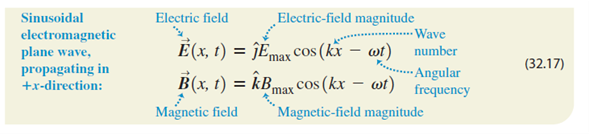# Problem: A sinusoidal electromagnetic wave having a magnetic field of amplitude 1.25 μT and a wavelength of 432 nm is traveling in the +-direction through empty space.Write the equations for the electric and magnetic fields as functions of x and t in the form of Eqs. (32.17).

###### FREE Expert Solution

Wave number:

$\overline{){\mathbf{k}}{\mathbf{=}}\frac{\mathbf{2}\mathbf{\pi }}{\mathbf{\lambda }}}$

Angular frequency:

$\overline{){\mathbf{\omega }}{\mathbf{=}}{\mathbf{2}}{\mathbf{\pi }}{\mathbf{f}}}$

k = 2π/λ = 2π/432 × 10-9  = 1.45 × 107 rad/m

1/λ = k/2π

ω = 2πf = 2π(c/λ) = 2π(ck/2π) = ck = 3.0 × 108 × 1.45 × 107  = 4.35 × 1015 rad/s###### Problem Details

A sinusoidal electromagnetic wave having a magnetic field of amplitude 1.25 μT and a wavelength of 432 nm is traveling in the +-direction through empty space.

Write the equations for the electric and magnetic fields as functions of x and t in the form of Eqs. (32.17).